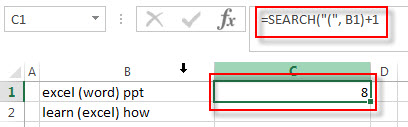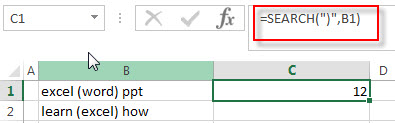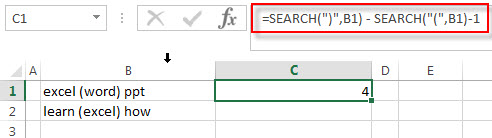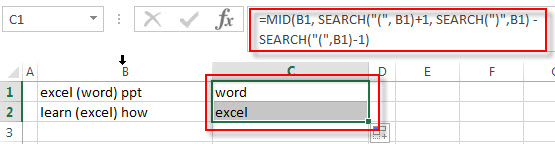# How to Extract Text between Parentheses

This post explains that how to extract text between the parentheses using the formula in excel.

## Extract text between parentheses

If you want to extract text between parentheses in a cell, then you can use the search function within the MID function to create a new excel formula.

For example, to extract data between parentheses in cell B1, you can write down the following formula using the MID function and the SEARCH function:

=MID(B1, SEARCH(“(“, B1)+1, SEARCH(“)”,B1) – SEARCH(“(“,B1)-1)

Let’s see how this formula works:

=MID()

The MID function used to extract a specific number of characters from a text string at a specific position. And this position value will be returned by the SEARCH function.

=SEARCH(“(“, B1)+1This search formula returns the position of the first left parentheses character in a text string in Cell B1, and then add 1, it will be the starting position that you want to extract text. It goes into the MID function as its start_num argument.

=SEARCH(“)”,B1)This formula returns the position of the first right parentheses character in a text string in Cell B1.

=SEARCH(“)”,B1) – SEARCH(“(“,B1)-1This formula returns the length of the text between parentheses. And the returned value will go into the MID function as its num_chars argument.

=MID(B1, SEARCH(“(“, B1)+1, SEARCH(“)”,B1) – SEARCH(“(“,B1)-1)

So far, we got the both start_num value and num_chars value, then the text between parentheses will be extracted by the MID function, see below screenshot:### Related Formulas

• Check If Cell Contains All Values from Range
If you want to check if a cell contains all values in a list, you can use a combination of the SEARCH function, the SUMPRODUCT function, the ISNUMBER function and COUNTA function…
• Extract Text between Brackets
If you want to extract text between brackets in a cell, you need to create a formula based on the SEARCH function and the MID function….

### Related Functions

• Excel SEARCH function
The Excel SEARCH function returns the number of the starting location of a substring in a text string.The syntax of the SEARCH function is as below:= SEARCH  (find_text, within_text,[start_num])…
• Excel MID function
The Excel MID function returns a substring from a text string at the position that you specify.The syntax of the MID function is as below:= MID (text, start_num, num_chars)…